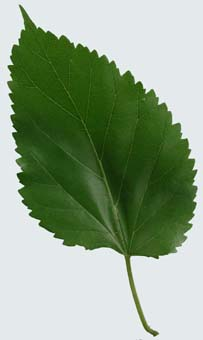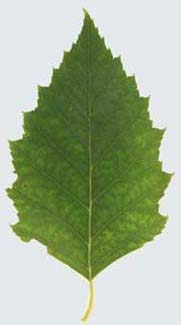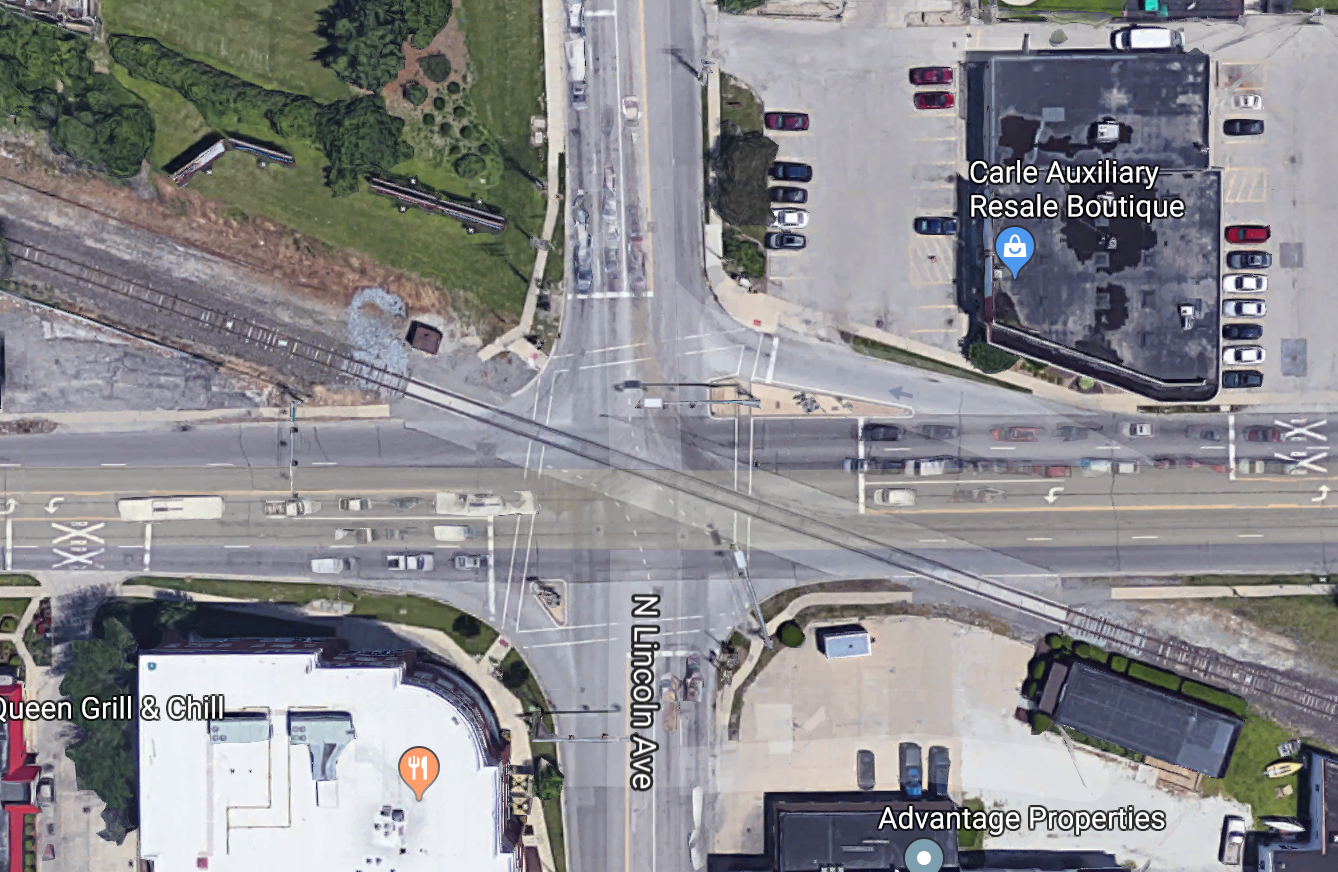CS 440/ECE 448
Margaret Fleck

## Motivation for statistical methods

Mulberry trees have edible berries and are critical for raising silkworms, The picture above shows a silk mill from a brief attempt (around 1834) to establish a silk industry in Northampton Massachusetts. But would you be able to tell a mulberry leaf from a birch leaf (below)? How would you even go about describing how they look different?Which is mulberry and which is birch?

These pictures come Guide to Practical Plants of New England (Pesha Black and Micah Hahn, 2004). This guide includes a variety of established descrete features for identifying plants, such as

• leaf shape is broad or narrow
• attachment to stem is opposite or alternate
• edge of leaf is smooth or toothed

Unfortunately, both of these leaves are broad, alternate, and toothed. But they look different.

These descrete features work moderately well for plants. However, modelling categories with discrete (logic-like) features doesn't generalize well to many real-world situations.

• too complex on realistic situations
• too much need for hand crafting representations
• too much need for expert knowledge

In practice, this means that logic models are incomplete and fragile A better approach is to learn models from examples, e.g. pictures, audio data, big text collections. However, this input data presents a number of challenges:

• It has uncertainty (e.g. from sensors).
• It has errors (e.g. "Department of Computer Silence").
• It is incomplete, often selectively so. E.g. we have much more training data for English than Zulu.
• It may lack annotation (e.g. explicit type labels).

So we use probabilistic models to capture what we know, and how well we know it.

## Discrete random variables

Suppose we're modelling the traffic light at University and Lincoln (two crossing streets plus a diagonal train track).Some useful random variables might be:

variable domain (possible values)
time {morning, afternoon, evening, night}
is-train {yes, no}
traffic-light {red, yellow, green}
barrier-arm {up, down}

Also exist continuous random variables, e.g. temperature (domain is all positive real numbers) or course-average (domain is [0-100]). We'll ignore those for now.

A state/event is represented by the values for all random variables that we care about.

## Probabilities

P(variable = value) or P(A) where A is an event

What percentage of the time does [variable] occur with [value]? E.g. P(barrier-arm = up) = 0.95

P(X=v and Y=w) or P(X=v,Y=w)

How often do we see X=v and Y=w at the same time? E.g. P(barrier-arm=up, time=morning) would be the probability that we see a barrier arm in the up position in the morning.

P(v) or P(v,w)

The author hopes that the reader can guess what variables these values belong to. For example, in context, it may be obvious that P(morning,up) is shorthand for P(barrier-arm=up, time=morning).

Probability notation does a poor job of distinguishing variables and values. So it is very important to keep an eye on types of variable and values, as well as the general context of what an author is trying to say. A useful heuristic is that

• variables are usually capital letters, and
• values are usually lowercase letters.

A distribution is an assignment of probability values to all events of interest, e.g. all values for particular random variable or pair of random variables.

## Properties of probabilities

The key mathematical properities of a probability distribution can be derived from Kolmogorov's axioms of probability:

0 $$\le$$ P(A)

P(True) = 1

P(A or B) = P(A) + P(B), if A and B are mutually exclusive events

It's easy to expand these three axioms into a more complete set of basic rules, e.g.

0 $$\le$$ P(A) $$\le$$ 1

P(True) = 1 and P(False) = 0

P(A or B) = P(A) + P(B) - P(A and B) [inclusion/exclusion, same as set theory]

If X has possible values p,q,r then P(X=p or X=q or X=r) = 1.

## Where do probabilities come from?

Probabilities can be estimated from direct observation of examples (e.g. large corpus of text data). Constraints on probabilities may also come from sceientific beliefs

• Theory connects observable probabilities to ones we can't observe. (e.g. icard access fails --> icard broken or access list wrong, because EngIT told me)
• The underlying model must have some simple form (e.g. virus infections grow exponentially) which can be used to fill in data from incomplete observations.
• Anything is possible, i.e. P(A) > 0. (We'll see smoothing techniques that fill zero values with small numbers.)
• Nothing is guaranteed, i.e. P(A) < 1.

The assumption that anything is possible is usually made to deal with the fact that our training data is incomplete, so we need to reserve some probability for all the possible events that we haven't happened to see yet.